# How to calculate percentage in Excel

In this article, we will learn How to calculate different percentages in Excel.

Percentages:

Calculating percentages is a basic task in calculating data. We surely need to know how much percentage of work is done. For example In school/collage we always calculated our attendance percentage carefully to be able to appear in exams. So calculating percentages is basic and anyone who works with numbers must know how to handle data in percentages. Generally, In mathematics if we need to multiply the result by 100 to get the percentage value but whereas Excel considers decimal as a percentage value. And you can change its formatting to percent by selecting the percent option in Home > % (symbol under Number section) as shown in the below snapshot.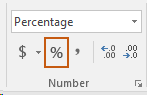Normal Percentage formula in Excel

Get the Percent for the sold product data in excel using a simple formula. Divide the sold product amount by the total amount of the product.

Syntax for the formula:

 Percent = Sold Amount / Total product

Types of percentage calculation in excel

• Total percentage
• Increase or Decrease in Percentage
• Profit, Loss and Discount Percentage
• Total from percentage

Example :

All of these might be confusing to understand. Let's understand how to use the function using an example. Here Let’s say you distributed your product in five regions of the country. You would like to know what percentage of total product is distributed in each region. See below image.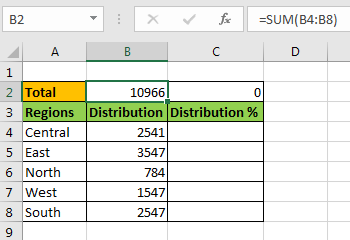I always prefer my total on the top of the table, so that whenever I extend the table I do not need to replace it. And it is always visible too if rows are frozen. So yeah it has its benefits.

Now to calculate percentage of total write this Percentage formula in cell C4 and drag it down:

Use the Formula

 =B4/\$B\$2

You will see fraction numbers. Now to convert them into percentage, select the cells and press CTRL + SHIFT+ %. This is a shortcut to convert numbers into percentage. Same thing can be done from the Number section of the Home tab.

Goto home tab

Click on % formatting in Number section.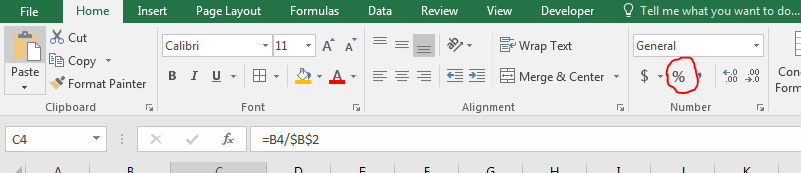And it’s done. We calculate the percentage of distribution in each region of Total distribution.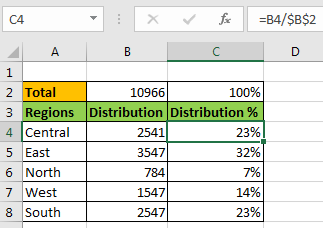Note :

we have given absolute reference of Total and relative reference of region. It will allow regions to change when copied in a relative cell but total will not change. In C2 we have some percentage, which is 100%. This can be used to validate the report. It should always be 100%. Here we use number formatting to add % symbol. In the background they are actually fraction numbers. 7% mean 0.7.

If you want your percentage in whole numbers then use this formula instead.

Use the formula

 =(B4/\$B\$2)*100

## Total Percentage

In the above example we used a total cell. Although you would like to have that total cell but in case you don’t, write this formula to calculate percentage of total.

 =B4 / SUM(\$B\$4:\$B\$8)

Here we just replaced the total cell with absolute function in that cell.

## Dynamic Percentage

The above examples are static. You will need to edit them if you extend your table. So how do you Calculate Percentage of Total Dynamically? We will use Tables here.

• Go to the Home tab.
• Click on “Format as Table”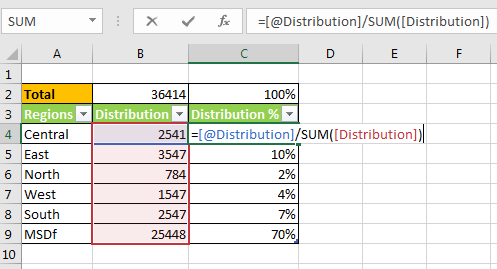Now each column is a dynamic named range. The headings are the name of ranges. This makes it very easy to read and write the formulas.

Increase or Decrease in Percentage change

Here we will use the increased percentage value formula to get the new Amount for the data set.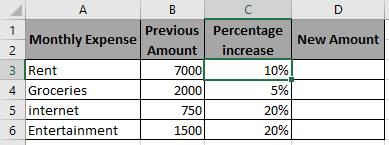Use the Formula in D3 cell

 =B3*(1+C3)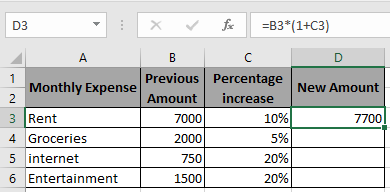As you can see in the above snapshot first New Amount after increment is 7700.

Copy the formula in the remaining cells to get the New Amount for the rest of the data.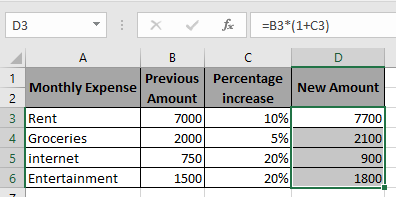New Amount can be calculated using the above procedure and understand how to handle with percentage decrease.

Here we have some products with their current Cost price and percentage decrement.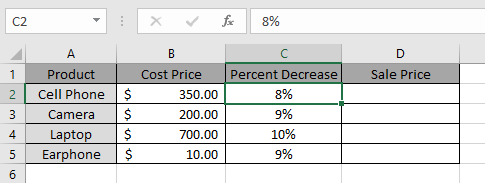And we need to find out the new discounted Price for the products.

Use the formula

 =B2*(1-C2)

B2 : Current Value

C2 : percentage change in Value

Explanation :

Value gets multiplied with (1 - C2) to get the discounted amount.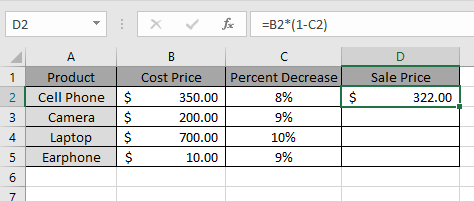Here the new Sales Price is listed in the Sales Price column.

Copy the formula to other cells using shortcut key Ctrl + D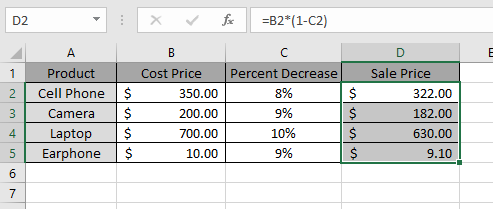This method can be used for various purposes. As you can see here the new Sales Price is listed in the Sales Price column.

## Profit, Loss and Discount Percentage

Here we will use the percentage value formula to get the percent value for the data.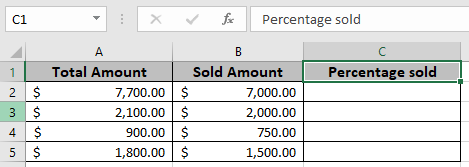Use the Formula in D3 cell

 = B2 / A2

Explanation : The sold amount will be divided by the total amount for a product.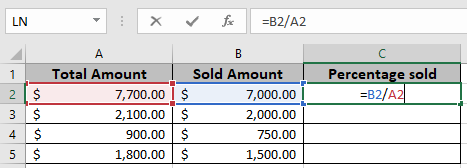Here the values to the function is given as cell reference.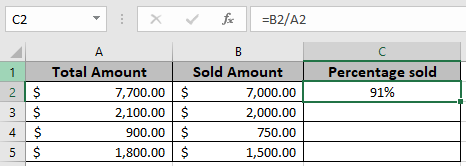As you can see in the above snapshot first old Amount before increment is 91% .

Copy the formula in the remaining cells using Ctrl + D shortcut, to get the New Amount for the rest of the values in the table.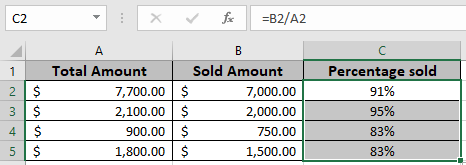In the above snapshot the mathematical formula is used to get the Percentage value for the sold product amount in excel.

Here we will use the above percentage discount formula in the below example.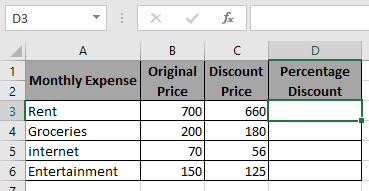Use the Formula in D3 cell

 =1-(C3/B3)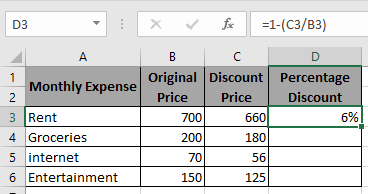As you can see in the above snapshot the first data percentage discount is 6%.

Copy the formula in the remaining cells to get the percentage discount for the rest of the data.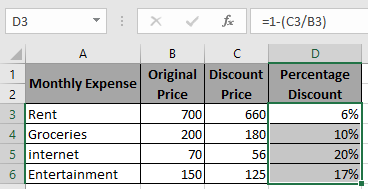Percentage discount can be calculated using the above method.

Profit margin percentage calculates the percentage change in profit margin using a simple mathematical operation shown below in the box.

Mathematical formula:

 Profit margin percentage = 1 - (cost price / sale price)

We will use this in Excel to find Percentage change in profit margin.

Let’s use the above formula on a few examples to learn better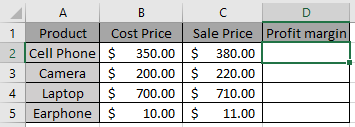Use the Formula in D2 cell

 =1-(B2/C2)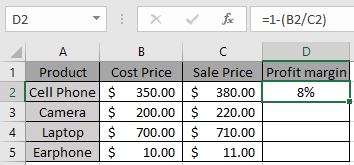As you can see in the above snapshot first data percentage of profit margin is 8%.

Copy the formula in the remaining cells to get the percentage change of profit margin for the rest of the data.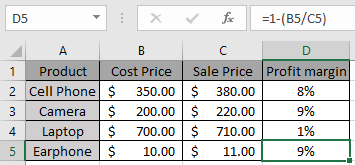Profit margin percent can be calculated using the above method.

How to get the total amount from Percentage?

Here we will use the basic mathematics observation used in excel. Excel considers percent value as decimal means 20% is 0.2 in excel. So we just need to divide the amount with percent value so as to get the total amount.

Generic formula:

 Total amount = discounted amount / discount percent

Discounted amount : amount after the discount applied

Discount percent : percent at which discount is given

Example:

All of these might be confusing to understand. So, let's test this formula via running it on the example shown below. Here we will use the generic formula to get the total value for the data.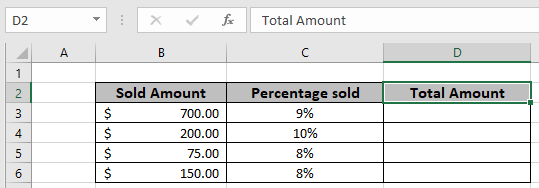Use the Formula in D3 cell

 = B3 / C3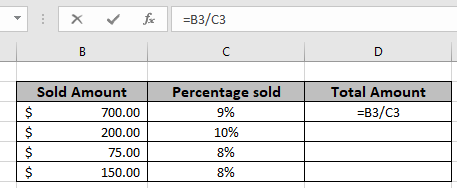The formula will be like as shown in the image above. Here the values to the function are given as cell reference.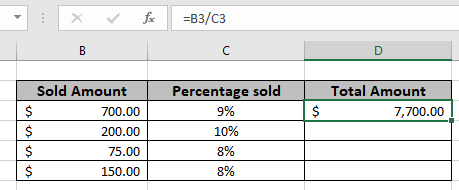As you can see in the above snapshot first old Amount comes out to be \$ 7,700. Copy the formula in the remaining cells using Ctrl + D shortcut, to get the New Amount for the rest of the values in the table.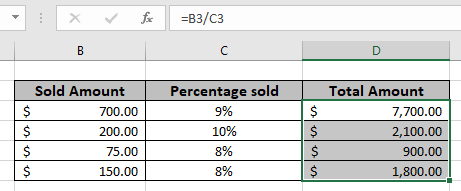In the above snapshot the mathematical formula is used to get the total value from the percent amount in excel.

Here are all the observational notes using the formula in Excel
Notes :

1. The function returns #VALUE error if any of the arguments is non numeric.
2. The function returns values for the negative as well as positive values.
3. Learn and Use the new formula to get the new amount having percentage values.

Hope this article about How to calculate percentages in Excel is explanatory. Find more articles on calculating values and related Excel formulas here. If you liked our blogs, share it with your friends on Facebook. And also you can follow us on Twitter and Facebook. We would love to hear from you, do let us know how we can improve, complement or innovate our work and make it better for you. Write to us at info@exceltip.com.

Related Articles:

Calculate Percentage of Total in Excel : Calculating percentages is a basic task in any field of work. We always want to know how much work is done. In school/collage we always calculated our attendance percentage carefully to be able to appear in the exam.

How to Calculate Percentage Discount in Excel : To calculate change in percentage discount of items, we simply subtract the discount price from total price and then get the percentage. This simple excel formula will get this done.

How to Increase by percentage in Excel :  To increase a given value by a given percentage we can simply add the percentage of increment in 100% and then multiply the actual number with it. This will increase the given value with the given percentage.

How to Calculate Profit margin percentage in Excel : To calculate the profit margin we simply divide the sales price by purchase price and subtract the result from 1. This gives us the percentage of profit margin.

Mathematical Percentage Decrease Change in Excel : In this article, how to use percentage decrease change formula in Excel 2016.We need to find the percentage decrease in value due to decrement percentage change.

How to Calculate Project Complete Percentage in Excel : When each task is completed, we say that project is completed 100%. In that case we would  like to monitor how the task is completed while we work on the project. In this article, we will learn how you can calculate the project complete percentage in Excel

Get the percentage for the sold product in Excel : In this article, we will learn about how to calculate the percent for the sold product in excel. Get the Percent for the sold product data in excel using a simple formula. Divide the sold product amount by the total amount of the product.

Find Total Amount From Percentage : for instance, working with percentage values in excel sometimes we need to get the total amount from the given percentage and the number. Given a discounted amount and discount percent, get the amount before discount or the total amount.

Popular Articles :